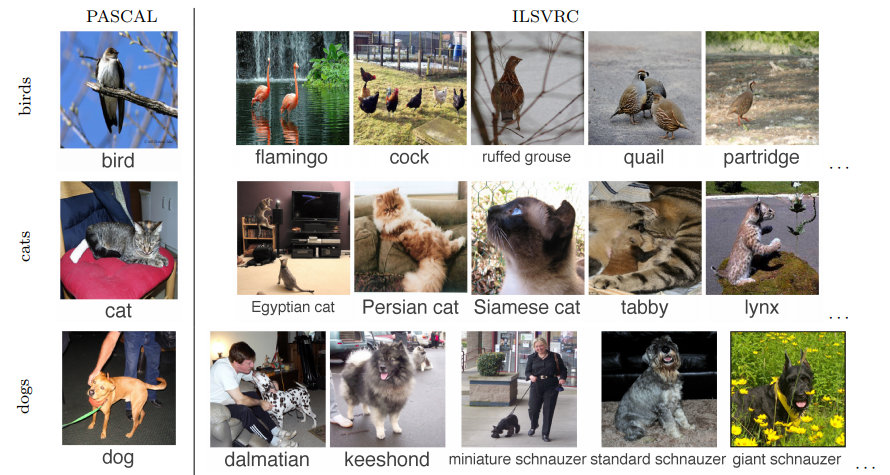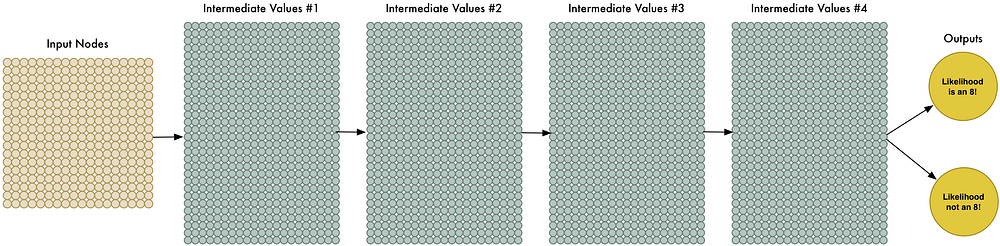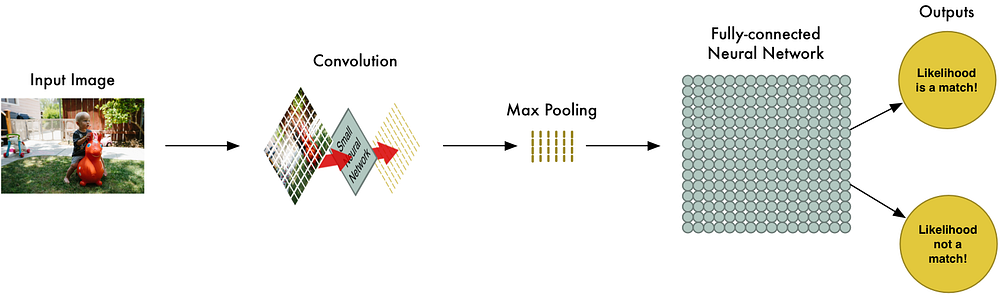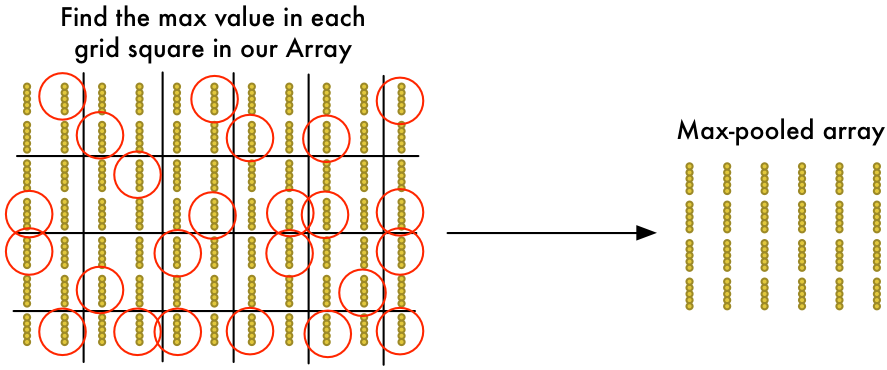Using Google’s Convolutional Neural Networks (CNN) for Image RecognitionConvolutional neural networks are the state of the art technique for image recognition-that is, identifying objects such as people or cars in pictures.We call this a “deep neural network” because it has more layers than a traditional neural network.How Convolution Works

Instead of feeding entire images into our neural network as one grid of numbers, we’re going to do something a lot smarter that takes advantage of the idea that an object is the same no matter where it appears in a picture.

Here’s how it’s going to work, step by step —Step 1: Break the image into overlapping image tiles

Similar to our sliding window search above, let’s pass a sliding window over the entire original image and save each result as a separate, tiny picture tile:

Step 2: Feed each image tile into a small neural network

Earlier, we fed a single image into a neural network to see if it was an “8”. We’ll do the exact same thing here, but we’ll do it for each individual image tile:

Step 3: Save the results from each tile into a new array

We don’t want to lose track of the arrangement of the original tiles. So we save the result from processing each tile into a grid in the same arrangement as the original image. It looks like this:

In other words, we’ve started with a large image and we ended with a slightly smaller array that records which sections of our original image were the most interesting.

Step 4: Downsampling

The result of Step 3 was an array that maps out which parts of the original image are the most interesting. But that array is still pretty big:The idea here is that if we found something interesting in any of the four input tiles that makes up each 2×2 grid square, we’ll just keep the most interesting bit. This reduces the size of our array while keeping the most important bits.

Final step: Make a prediction

So far, we’ve reduced a giant image down into a fairly small array.

Guess what? That array is just a bunch of numbers, so we can use that small array as input into another neural network. This final neural network will decide if the image is or isn’t a match. To differentiate it from the convolution step, we call it a “fully connected” network.

Our image processing pipeline is a series of steps: convolution, max-pooling, and finally a fully-connected network.

When solving problems in the real world, these steps can be combined and stacked as many times as you want! You can have two, three or even ten convolution layers. You can throw in max pooling wherever you want to reduce the size of your data.

The basic idea is to start with a large image and continually boil it down, step-by-step, until you finally have a single result. The more convolution steps you have, the more complicated features your network will be able to learn to recognize.

In machine learning, having more data is almost always more important that having better algorithms.Now you know why Google is so happy to offer you unlimited photo storage. They want your sweet, sweet data!

TFlearn is a wrapper around Google’s TensorFlow deep learning library that exposes a simplified API. It makes building convolutional neural networks as easy as writing a few lines of code to define the layers of our network.

 # -*- coding: utf-8 -*- “”” Based on the tflearn example located here: https://github.com/tflearn/tflearn/blob/master/examples/images/convnet_cifar10.py “”” from __future__ import division, print_function, absolute_import # Import tflearn and some helpers import tflearn from tflearn.data_utils import shuffle from tflearn.layers.core import input_data, dropout, fully_connected from tflearn.layers.conv import conv_2d, max_pool_2d from tflearn.layers.estimator import regression from tflearn.data_preprocessing import ImagePreprocessing from tflearn.data_augmentation import ImageAugmentation import pickle # Load the data set X, Y, X_test, Y_test = pickle.load(open(“full_dataset.pkl“, “rb“)) # Shuffle the data X, Y = shuffle(X, Y) # Make sure the data is normalized img_prep = ImagePreprocessing() img_prep.add_featurewise_zero_center() img_prep.add_featurewise_stdnorm() # Create extra synthetic training data by flipping, rotating and blurring the # images on our data set. img_aug = ImageAugmentation() img_aug.add_random_flip_leftright() img_aug.add_random_rotation(max_angle=25.) img_aug.add_random_blur(sigma_max=3.) # Define our network architecture: # Input is a 32×32 image with 3 color channels (red, green and blue) network = input_data(shape=[None, 32, 32, 3], data_preprocessing=img_prep, data_augmentation=img_aug) # Step 1: Convolution network = conv_2d(network, 32, 3, activation=‘relu‘) # Step 2: Max pooling network = max_pool_2d(network, 2) # Step 3: Convolution again network = conv_2d(network, 64, 3, activation=‘relu‘) # Step 4: Convolution yet again network = conv_2d(network, 64, 3, activation=‘relu‘) # Step 5: Max pooling again network = max_pool_2d(network, 2) # Step 6: Fully-connected 512 node neural network network = fully_connected(network, 512, activation=‘relu‘) # Step 7: Dropout – throw away some data randomly during training to prevent over-fitting network = dropout(network, 0.5) # Step 8: Fully-connected neural network with two outputs (0=isn’t a bird, 1=is a bird) to make the final prediction network = fully_connected(network, 2, activation=‘softmax‘) # Tell tflearn how we want to train the network network = regression(network, optimizer=‘adam‘, loss=‘categorical_crossentropy‘, learning_rate=0.001) # Wrap the network in a model object model = tflearn.DNN(network, tensorboard_verbose=0, checkpoint_path=‘bird-classifier.tfl.ckpt‘) # Train it! We’ll do 100 training passes and monitor it as it goes. model.fit(X, Y, n_epoch=100, shuffle=True, validation_set=(X_test, Y_test), show_metric=True, batch_size=96, snapshot_epoch=True, run_id=‘bird-classifier‘) # Save model when training is complete to a file model.save(“bird-classifier.tfl“) print(“Network trained and saved as bird-classifier.tfl!“)

How to Build a Convolutional Neural Network?

Building a CNN from scratch can be an expensive and time–consuming undertaking. Having said that, a number of APIs have been developed recently developed that aim to enable the organizations to glean insights without the need of in-house machine learning or computer vision expertise.

Google Cloud Vision is the visual recognition API of Google and uses a REST API. It is based on the open-source TensorFlow framework. It detects the individual faces and objects and contains a pretty comprehensive label set.

IBM Watson Visual Recognition

IBM Watson Visual Recognition is a part of the Watson Developer Cloud and comes with a huge set of built-in classes but is built really for training custom classes based on the images you supply. It also supports a number of nifty features including NSFW and OCR detection like Google Cloud Vision.

Clarif.ai

Clarif.ai is an upstart image recognition service that also utilizes a REST API. One interesting aspect regarding Clarif.ai is that it comes with a number of modules that are helpful in tailoring its algorithm to specific subjects such as food, travel and weddings.

While the above APIs are suitable for few general applications, you might still be better off developing a custom solution for specific tasks. Fortunately, a number of libraries are available that make the lives of developers and data scientists a little easier by dealing with the optimization and computational aspects allowing them to focus on training models. Many of these libraries including Theano, Torch, DeepLearning4J and TensorFlow have been successfully used in a wide variety of applications.

An Interesting Application of Convolutional Neural Networks

Adding Sounds to Silent Movies Automatically